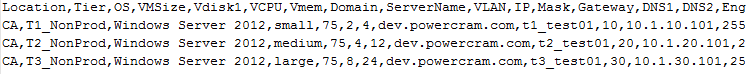## Thursday, May 14, 2015

### Use PowerShell to Save Excel Worksheet as CSV

I'm working on a PowerShell script to launch some virtual servers on VMWare based on input from a CSV file. To create the CSV file I'm using Excel, and I have more than one worksheet in the Excel workbook. Since it's a bit of a hassle to use Excel's GUI to save a given worksheet as a CSV, especially when this has to be done more than once, I set out to find a way to do this programatically. At first I was indifferent on using either a macro within Excel, or a PowerShell method. I decided on PowerShell because I'm already using a PS script to launch the servers, so I decided I would write a block of code to open my spreadsheet and create a CSV from the desired worksheet.Excel spreadsheet file.
In my quest to figure this out I ran across a lot of pieces, but not a complete solution, and as is often the case on the web I couldn't find explanations of some of the solutions. That's what prompted me to save this all here. I'll step through some of the logic, then post the script in its entirety at the bottom. The best resource I found, although not complete (for my needs), was this great post from LazyWinAdmin.

First thing is to set some variables specific to my environment, the path and name to both my input (xlsx) and output (csv) files, and the name of the tab or worksheet in the spreadsheet.
```\$Sheet = "CreateVM-CA"
\$xls = "C:\Temp\PC\PCTest.xlsx"
\$csv = "C:\Temp\PC\\$Sheet.csv"```

NOTE: Make sure you save your Excel file as this script reads the file from disk & not what's in an open workbook.

Next we will open Excel, the workbook, and the specific worksheet we want to export. I'm also using "Excel.Visible = \$False" to prevent the Excel GUI from opening, and "Excel.DisplayAlerts = \$False" to prevent messages such as being prompted to overwrite the file if it already exists (see below).
```\$objExcel = New-Object -ComObject Excel.Application
\$objExcel.Visible = \$False
\$WorkBook = \$objExcel.Workbooks.Open(\$xls)
\$WorkSheet = \$WorkBook.sheets.item("\$Sheet")```Prompted to replace existing file (when not using DisplayAlerts=\$False)
A useful note (again thanks to LazyWinAdmin) is that we can verify excel has opened, and we are working with the desired workbook using the following, which lists the available worksheets within the opened workbook.
`\$WorkBook.sheets | Select-Object -Property Name`Listing Excel worksheets with PowerShell.
Saving the file. When saving the file we will, of course specify that it is a CSV, but also the file type (CSV) is designated with the number 6. For some reason you can't pass the number 6 directly in the SaveAs command, so we are using the variable xlCSV. In my discovery I ran across a list of several file types, but cannot find that now. It's out there somewhere....
```\$xlCSV = 6
\$WorkBook.SaveAs(\$csv,\$xlCSV)```

Closing/quitting Excel. Initially I was using just the first command to quit Excel. However, I discovered that it remained open and subsequent executions of my script would fail to write the file because the open Excel process had the file locked. So after a little research and testing I found the second command which kills the process, and prevents the CSV file from being locked.
```\$objExcel.quit()
[System.Runtime.Interopservices.Marshal]::ReleaseComObject(\$objExcel)```

This little script works beautifully now and does just what I need by opening a specific worksheet from an Excel workbook, then saving it as a CSV file. Here's the entire script.
```\$Sheet = "CreateVM-CA"
\$xls = "C:\Temp\PC\PCTest.xlsx"
\$csv = "C:\Temp\PC\\$Sheet.csv"
\$objExcel = New-Object -ComObject Excel.Application
\$objExcel.Visible = \$False
\$WorkBook = \$objExcel.Workbooks.Open(\$xls)
\$WorkSheet = \$WorkBook.sheets.item("\$Sheet")
\$xlCSV = 6
\$WorkBook.SaveAs(\$csv,\$xlCSV)
\$objExcel.quit()
[System.Runtime.Interopservices.Marshal]::ReleaseComObject(\$objExcel)```
```
```Output CSV file.

1.Thanks for this. I found the list of file type constants at https://msdn.microsoft.com/en-us/library/office/ff198017.aspx

2.+1
you just saved me countless hours of noodling....

3.\$WorkBook.SaveAs(\$csv,\$xlCSV) should be \$WorkSheet.SaveAs(\$csv,\$xlCSV) yeah?

1.Great point Noeffinway99. The \$WorkBook.SaveAs(\$csv,\$xlCSV) works as long as there's only one worksheet (which is typically the case with a CSV), however, if there are multiple worksheets then \$WorkSheet.SaveAs(\$csv,\$xlCSV) would be the way to go.